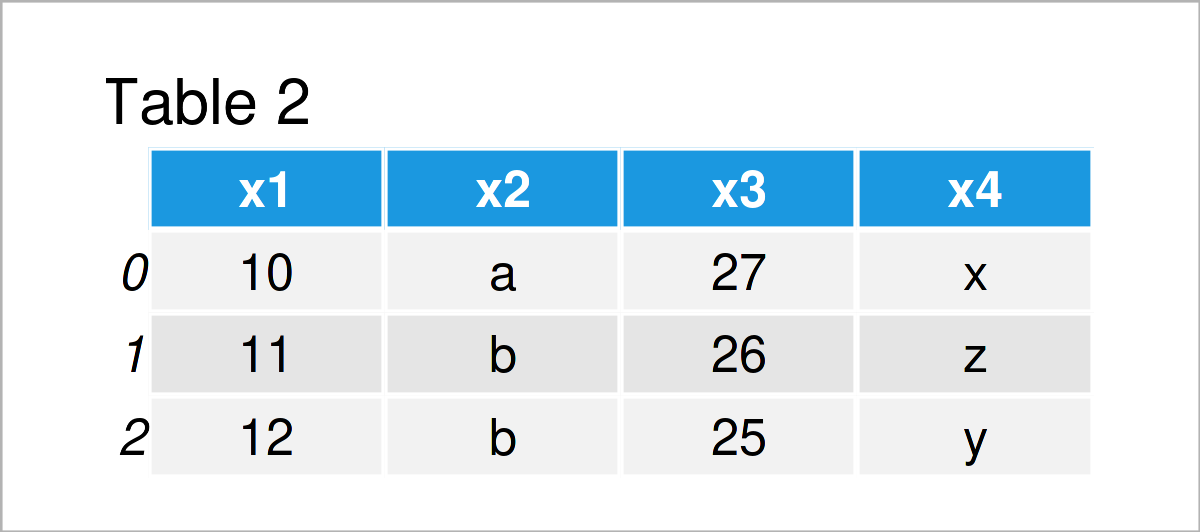# Slice pandas DataFrame by Index in Python (Example)

This tutorial illustrates how to split a pandas DataFrame at a certain row index position in the Python programming language.

The tutorial consists of these contents:

Let’s get started…

## Example Data & Add-On Libraries

We first need to import the pandas library:

`import pandas as pd                         # Load pandas`

As a next step, I’ll also need to create some data that we can use in the exemplifying code below:

```data = pd.DataFrame({'x1':range(10, 18),    # Create pandas DataFrame
'x2':['a', 'b', 'b', 'c', 'd', 'a', 'b', 'd'],
'x3':range(27, 19, - 1),
'x4':['x', 'z', 'y', 'y', 'x', 'y', 'z', 'x']})
print(data)                                 # Print pandas DataFrame```As you can see based on Table 1, the exemplifying data is a pandas DataFrame containing eight rows and four columns.

## Example: Split pandas DataFrame at Certain Index Position

This example explains how to divide a pandas DataFrame into two different subsets that are split at a particular row index.

For this, we first have to define the index location at which we want to slice our data set (i.e. 3):

```split_point = 3                             # Define split point
print(split_point)                          # Print split point
# 3```

In the next step, we can use this splitting point to extract all rows before this index point:

```data_upper = data.iloc[:split_point]        # Create upper data set
print(data_upper)                           # Print DataFrame of upper rows```After executing the previous syntax the pandas DataFrame subset shown in Table 2 has been created.

Next, we can use our splitting point once again to select only the rows below the splitting point:

```data_lower = data.iloc[split_point:]        # Create lower data set
print(data_lower)                           # Print DataFrame of lower rows```Table 3 shows the output of the previous Python programming syntax – A second pandas DataFrame subset containing all rows below the slicing point.

## Video & Further Resources

I have recently released a video on my YouTube channel, which illustrates the Python code of this tutorial. You can find the video below.

Please accept YouTube cookies to play this video. By accepting you will be accessing content from YouTube, a service provided by an external third party.If you accept this notice, your choice will be saved and the page will refresh.

Also, you could read some related tutorials on this website. A selection of tutorials is shown below:

This article has explained how to slice a pandas DataFrame at a specific row index position in the Python programming language. If you have any further comments and/or questions, please let me know in the comments section.

Subscribe to the Statistics Globe Newsletter